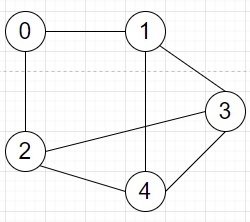# Program to check whether odd length cycle is in a graph or not in Python

Suppose we have an undirected graph we have to check whether we can find an odd length cycle inside it or not.

So, if the input is like adj_list = [[1, 2], [0, 3, 4], [0, 3, 4], [1, 2, 4], [1, 2, 3]]then the output will be True as there are odd length cycles like [0, 1, 3, 4, 2], [1, 3, 4], [2, 3, 4].

To solve this, we will follow these steps −

• Define a function dfs() . This will take node, i
• if node is in path, then
• return true when (i - path[node]) is odd
• if node is visited, then
• return False
• mark node as visited
• path[node] := i
• for each c in arr[node], do
• if dfs(c, i + 1) is true, then
• return True
• del path[node]
• return False
• From the main method do the following −
• visited := a new set, path :=a new map
• for i in range 0 to size of arr, do
• if dfs(i, 0) is true, then
• return True
• return False

## Example (Python)

Let us see the following implementation to get better understanding −

Live Demo

class Solution:
def solve(self, arr):
def dfs(node, i):
if node in path:
return (i - path[node]) % 2 == 1
if node in visited:
return False
path[node] = i
for c in arr[node]:
if dfs(c, i + 1):
return True
del path[node]
return False
visited, path = set(), {}
for i in range(len(arr)):
if dfs(i, 0):
return True
return False
ob = Solution()
adj_list = [[1, 2], [0, 3, 4], [0, 3, 4], [1, 2, 4], [1, 2, 3]]
print(ob.solve(adj_list))

## Input

[[1, 2], [0, 3, 4], [0, 3, 4], [1, 2, 4], [1, 2, 3]]

## Output

True